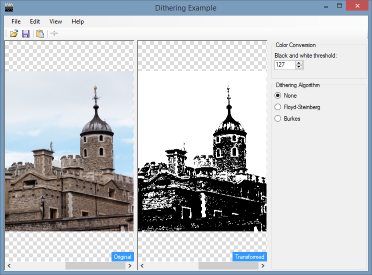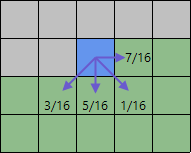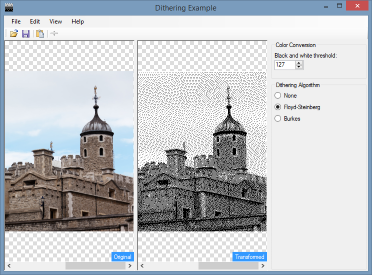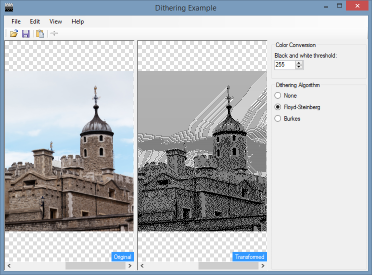## Dithering an image using the Floyd‑Steinberg algorithm in C#

In my previous introductory post, I briefly described the concept of dithering an image. In this article, I will describe how to dither an image in C# using the Floyd–Steinberg algorithm.

### The Demo Application

For this series of articles, I'll be using the same demo application, the source of which can be found on GitHib. There's a few things about the demo I wish to cover before I get onto the actual topic of dithering.

Algorithms can be a tricky thing to learn about, and so I don't want the demo to be horribly complicated by including a additional complex code unrelated to dithering. At the same time, bitmap operations are expensive, so there is already some advanced code present.

As I mentioned in my introduction, dithering is part of a process. For this demo, the process will be converting a 32bit image into a 1bit image as this is the simplest conversion I can stick in a demo. This does not mean that the dithering techniques can only be used to convert an image to black and white, it is simply to make the demo easier to understand.

I have however broken this rule when it comes to the actual image processing. The .NET `Bitmap` object offers `SetPixel` and `GetPixel` methods. You should try and avoid using these as they will utterly destroy the performance of whatever it is you are trying to do. The best way of accessing pixel data is to access it directly using `Bitmap.LockBits`, pointer manipulation, then `Bitmap.UnlockBits`. In this demo, I use this approach to create a custom array of colours, and while it is very fast, if you want better performance it is probably better to manipulate individual bytes via pointers. However, this requires much more complex code to account for different colour depths and is well beyond the scope of this demo.

I did a version of the demo program using `SetPixel` and `GetPixel`. Saying it was slow was an understatement. Just pretend these methods don't exist!

### Converting a colour to black or white

In order to convert the image to 2 colours, I scan each pixel and convert it to grayscale. If the grayscale value is around 50% (127 in .NET's 0 - 255 range), then the transformed pixel will be black, otherwise it will be white.

``````byte gray;

gray = (byte)(0.299 * pixel.R + 0.587 * pixel.G + 0.114 * pixel.B);

return gray < 128 ? new ArgbColor(pixel.A, 0, 0, 0) : new ArgbColor(pixel.A, 255, 255, 255);``````

This actually creates quite a nice result from our demonstration image, but results will vary depending on the image.### Floyd‑Steinberg dithering

The Floyd‑Steinberg algorithm is an error diffusion algorithm, meaning for each pixel an "error" is generated and then distributed to four pixels around the surrounding the current pixel. Each of the four offset pixels has a different weight - the error is multiplied by the weight, divided by 16 and then added to the existing value of the offset pixel.

As a picture is definitely worth a thousand words, the diagram below shows the weights.• 7 for the pixel to the right of the current pixel
• 3 for the pixel below and to the left
• 5 for the pixel below
• 1 for the pixel below and to the right

### Calculating the error

The error calculation in our demonstration program is simple, although in actuality it's 3 errors, one for the red, green and blue channels. All we are doing is taking the difference between the channels transformed value from the original value.

``````redError = originalPixel.R - transformedPixel.R;
blueError = originalPixel.G - transformedPixel.G;
greenError = originalPixel.B - transformedPixel.B;``````

### Applying the error

Once we have our error, it's just a case of getting each neighbouring pixels to adjust, and applying each error the appropriate channel. The `ToByte` extension method in the snippet below simply converts the calculated integer to a byte, while ensuring it is in the 0-255 range.

``````offsetPixel.R = (offsetPixel.R + ((redError * 7) >> 4)).ToByte();
offsetPixel.G = (offsetPixel.G + ((greenError * 7) >> 4)).ToByte();
offsetPixel.B = (offsetPixel.B + ((blueError * 7) >> 4)).ToByte();``````

#### Bit shifting for division

As 16 is a power of two, it means we can use bit shifting to do the division. While this may be slightly less readable if you aren't hugely familiar with it, it ought to be faster. I did a quick benchmark test using a sample of 1 million, 10 million and then 100 million random numbers. Using bit shifting to divide each sample by 16 took roughly two thirds of the time it took to do the same sets with integer division. This is probably a useful thing to know when performing thousands of operations processing an image.

### Dithering a single pixel

Here's the code used by the demonstration program to dither a single source pixel - the `ArbColor` data representing each pixel is stored in a one-dimensional array using row-major order.

``````ArgbColor offsetPixel;
int redError;
int blueError;
int greenError;
int offsetIndex;
int index;

index = y * width + x;
redError = originalPixel.R - transformedPixel.R;
blueError = originalPixel.G - transformedPixel.G;
greenError = originalPixel.B - transformedPixel.B;

if (x + 1 < width)
{
// right
offsetIndex = index + 1;
offsetPixel = original[offsetIndex];
offsetPixel.R = (offsetPixel.R + ((redError * 7) >> 4)).ToByte();
offsetPixel.G = (offsetPixel.G + ((greenError * 7) >> 4)).ToByte();
offsetPixel.B = (offsetPixel.B + ((blueError * 7) >> 4)).ToByte();
original[offsetIndex] = offsetPixel;
}

if (y + 1 < height)
{
if (x - 1 > 0)
{
// left and down
offsetIndex = index + width - 1;
offsetPixel = original[offsetIndex];
offsetPixel.R = (offsetPixel.R + ((redError * 3) >> 4)).ToByte();
offsetPixel.G = (offsetPixel.G + ((greenError * 3) >> 4)).ToByte();
offsetPixel.B = (offsetPixel.B + ((blueError * 3) >> 4)).ToByte();
original[offsetIndex] = offsetPixel;
}

// down
offsetIndex = index + width;
offsetPixel = original[offsetIndex];
offsetPixel.R = (offsetPixel.R + ((redError * 5) >> 4)).ToByte();
offsetPixel.G = (offsetPixel.G + ((greenError * 5) >> 4)).ToByte();
offsetPixel.B = (offsetPixel.B + ((blueError * 5) >> 4)).ToByte();
original[offsetIndex] = offsetPixel;

if (x + 1 < width)
{
// right and down
offsetIndex = index + width + 1;
offsetPixel = original[offsetIndex];
offsetPixel.R = (offsetPixel.R + ((redError * 1) >> 4)).ToByte();
offsetPixel.G = (offsetPixel.G + ((greenError * 1) >> 4)).ToByte();
offsetPixel.B = (offsetPixel.B + ((blueError * 1) >> 4)).ToByte();
original[offsetIndex] = offsetPixel;
}
}``````

Much of the code is duplicated, with a different co-efficient for the multiplication, and (importantly!) guards to skip pixels when the current pixel is either the first or last pixel in the row, or is within the final row.

### And the result?

The image below shows our sample image dithered using the Floyd–Steinberg algorithm. It doesn't look too bad!By changing the threshold at which colours are converted to black or white, we can affect the output of the dithering even if the conversion is to solid black.(Note: The thumbnail hasn't resized well, the actual size version looks better)

### Source Code

The latest source code for this demonstration (which will be extended over time to include additional algorithms) can be found at our GitHib page.

The source code from the time this article was created is available from the link below, however may not be fully up to date.

## Related articles you may be interested in

Filename Description Version Release Date
DitheringTest.zip
• md5: 960d00ff8054b8711bf56c562a141126

Sample C# project demonstrating the Floyd‑Steinberg and Burkes algorithms for dithering an image.

1.0.0.0 06/06/2015

While we appreciate comments from our users, please follow our posting guidelines. Have you tried the Cyotek Forums for support from Cyotek and the community?

Styling with Markdown is supported